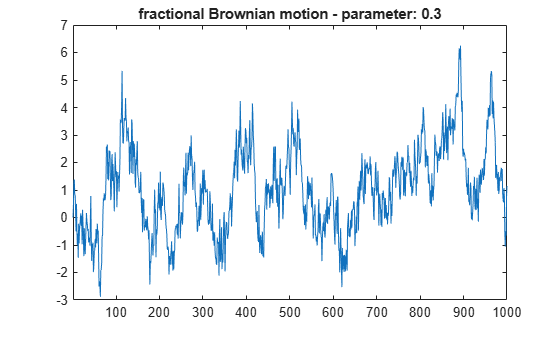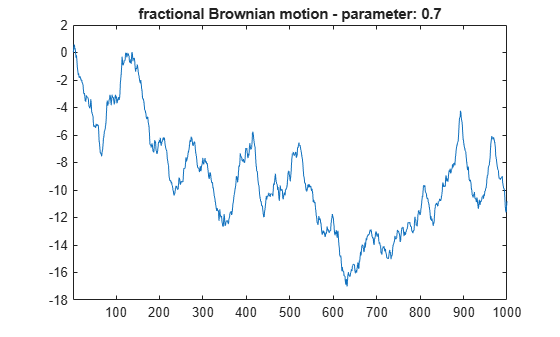Main Content

# 1-D Fractional Brownian Motion Synthesis

This example shows how to generate a fractional Brownian motion signal using the `wfbm` function.

A fractional Brownian motion (`fBm`) is a continuous-time Gaussian process depending on the Hurst parameter 0 < `H` < 1. It generalizes the ordinary Brownian motion corresponding to `H` = 0.5 and whose derivative is white noise. The `fBm` is self-similar in distribution and the variance of the increments is given by

`Var(fBm(t) - fBm(s)) = v abs(t-s)^(2H)`,

where v is a positive constant. The `fBm` exhibits long-range dependence for `H` > 0.5 and short or intermediate dependence for `H` < 0.5.

For purposes of reproducibility, set the random seed to the default value. Generate fractional Brownian motion with length 1000 for `H` = 0.3. Plot the result.

```rng default H = 0.3; len = 1000; fBm03 = wfbm(H,len,'plot');```Generate fractional Brownian motion with length 1000 for `H` = 0.7. Plot the result. Because `H` > 0.5, the fractional Brownian motion exhibits a stronger low-frequency component and has, locally, less irregular behavior.

```rng default H = 0.7; fBm07 = wfbm(H,len,'plot');```Confirm the previous syntax is equivalent to generating fractional Brownian motion using the orthogonal `db10` wavelet and six reconstruction steps.

```rng default w = 'db10'; ns = 6; fBm07x = wfbm(H,len,w,ns); max(abs(fBm07-fBm07x))```
```ans = 0 ```

## SupportGet trial now